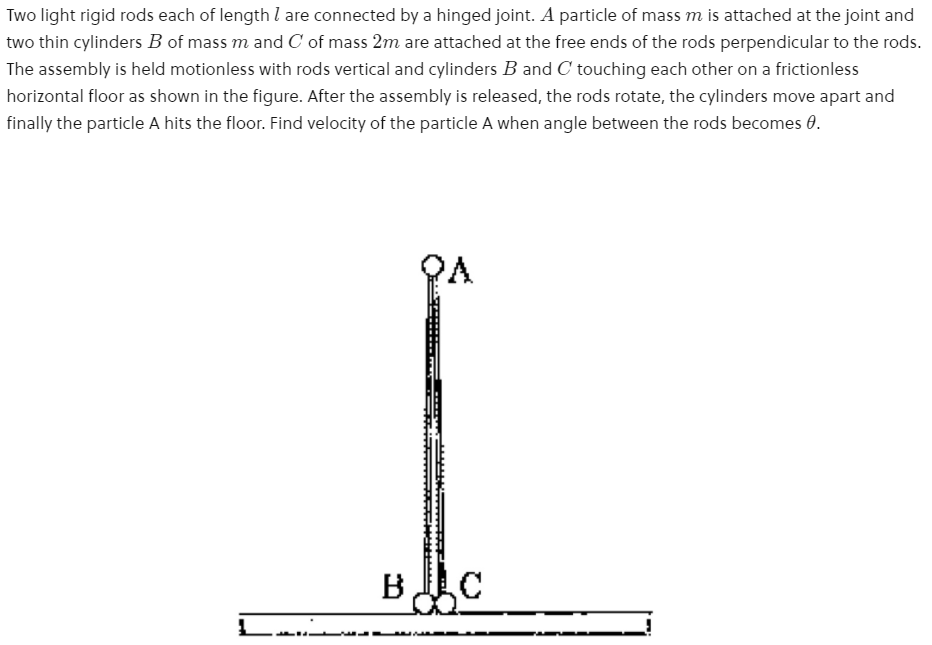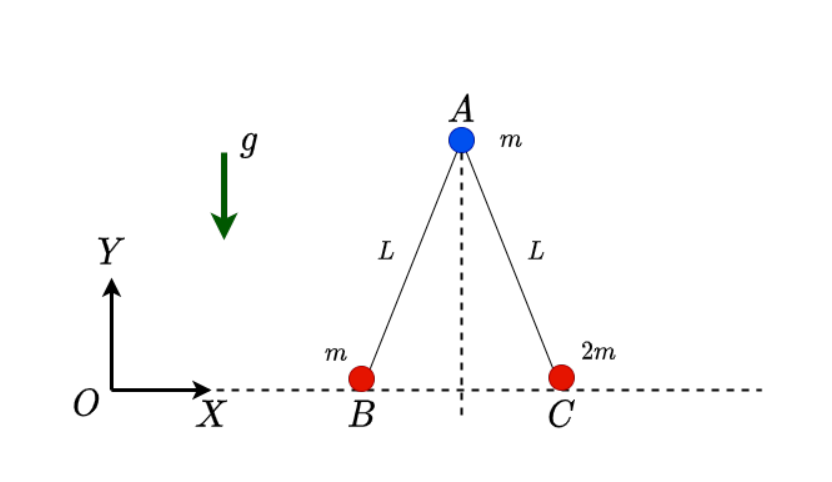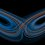# An Interesting Mechanics Problem - My Attempt

Recently, the following problem was shared in the Brilliant Community:My attempt is as follows. Consider the diagram at an arbitrary instant as shown below.

Let:

$\angle BAC = 2\phi$Say the mass $B$ is situated at a distance $s$ along the X-axis. Then the coordinates of each mass are:

$x_B = s$ $y_B = 0$

$x_A = s + L\sin{\phi}$ $y_A = L\cos{\phi}$

$x_C = s + 2L\sin{\phi}$ $y_C = 0$

Since no external force acts one the system along the X direction, the momentum along X is conserved. This gives:

$m\dot{x}_A + m\dot{x}_B + 2m\dot{x}_C = 0$

The initial momentum of the system is zero. Plugging in all variables above and simplifying gives:

$\dot{s} = -\frac{5}{4}L \dot{\phi} \cos{\phi} \dots (1)$

The kinetic energy of this system is:

$\mathcal{T} = \frac{m}{2}\left(\dot{x}_A^2 + \dot{y}_A^2\right) + \frac{m}{2}\left(\dot{x}_B^2 + \dot{y}_B^2\right) + \frac{2m}{2}\left(\dot{x}_C^2 + \dot{y}_C^2\right)$

Simplifying the above expression gives:

$\mathcal{T} = 2m\dot{s}^2 + 5mL\dot{s} \dot{\phi}\cos{\phi} + \frac{mL^2\dot{\phi}^2}{2} + 4mL^2\cos^2{\phi} \dot{\phi}^2$

Eliminating $\dot{s}$ by using (1) and simplifying gives:

$\mathcal{T} = \frac{7mL^2\dot{\phi}^2\cos^2{\phi}}{8} + \frac{mL^2\dot{\phi}^2}{2}$

The potential energy of the system is:

$\mathcal{V} = mgy_A + mgy_B + 2mgy_C$ $\mathcal{V} = mgL\cos{\phi}$

Initial potential energy is:

$\mathcal{V}_o = mgL$

Initial kinetic energy is:

$\mathcal{T}_o = 0$

$\phi(0)=0$ $\dot{\phi}(0) = 0$

Applying conservation of energy gives:

$\mathcal{T} + \mathcal{V} = \mathcal{T}_o + \mathcal{V}_o$ $\implies \frac{7mL^2\dot{\phi}^2\cos^2{\phi}}{8} + \frac{mL^2\dot{\phi}^2}{2} + mgL\cos{\phi} = mgL$

$\implies \dot{\phi} = \sqrt{\frac{g(1-\cos{\phi})}{\frac{7L\cos^2{\phi}}{8} + \frac{L}{2}}}$

Now, when the angle between the rods is 30 degrees, then:

$\phi = \frac{\pi}{12}$

This gives the value of $\dot{\phi}$ at that instant.

Finally:

$x_A = s + L\sin{\phi}$ $y_A = L\cos{\phi}$

$\dot{x}_A = \dot{s} + L\cos{\phi}\dot{\phi} = -\frac{L\cos{\phi} \dot{\phi}}{4}$ $\dot{y}_A = -L\sin{\phi} \dot{\phi}$

The velocity of point $A$ at this instant :

$\vec{v}_A = \dot{x}_A \ \hat{i} + \dot{y}_A \ \hat{j}$

The final expression for speed is:

$\boxed{V = \sqrt{\frac{gL}{2}\left(1 - \cos{\phi}\right)\left(\frac{1 + 15\sin^2{\phi}}{7\cos^2{\phi}+4}\right)}}$

Assumptions:

• The cylinders are treated as point masses.Note by Karan Chatrath
10 months, 2 weeks ago

This discussion board is a place to discuss our Daily Challenges and the math and science related to those challenges. Explanations are more than just a solution — they should explain the steps and thinking strategies that you used to obtain the solution. Comments should further the discussion of math and science.

When posting on Brilliant:

• Use the emojis to react to an explanation, whether you're congratulating a job well done , or just really confused .
• Ask specific questions about the challenge or the steps in somebody's explanation. Well-posed questions can add a lot to the discussion, but posting "I don't understand!" doesn't help anyone.
• Try to contribute something new to the discussion, whether it is an extension, generalization or other idea related to the challenge.

MarkdownAppears as
*italics* or _italics_ italics
**bold** or __bold__ bold
- bulleted- list
• bulleted
• list
1. numbered2. list
1. numbered
2. list
Note: you must add a full line of space before and after lists for them to show up correctly
paragraph 1paragraph 2

paragraph 1

paragraph 2

[example link](https://brilliant.org)example link
> This is a quote
This is a quote
    # I indented these lines
# 4 spaces, and now they show
# up as a code block.

print "hello world"
# I indented these lines
# 4 spaces, and now they show
# up as a code block.

print "hello world"
MathAppears as
Remember to wrap math in $$ ... $$ or $ ... $ to ensure proper formatting.
2 \times 3 $2 \times 3$
2^{34} $2^{34}$
a_{i-1} $a_{i-1}$
\frac{2}{3} $\frac{2}{3}$
\sqrt{2} $\sqrt{2}$
\sum_{i=1}^3 $\sum_{i=1}^3$
\sin \theta $\sin \theta$
\boxed{123} $\boxed{123}$

Sort by:

@Lil Doug This is my attempt. Unable to tag KrishnaKarthik. Do tag him if possible

- 10 months, 2 weeks ago

Hey; very nice approach. So this is what momentum is used for, outside of collisions, of course. Nice.

- 10 months, 2 weeks ago

Suppose that the horizontal displacement of $A$ from its starting position is $x$, and that the angle $\angle BAC = 2\theta$. Then $A,B,C$ have position vectors $\binom{x}{L\cos\theta} \hspace{2cm} \binom{x - L\sin\theta}{0} \hspace{2cm} \binom{x+L\sin\theta}{0}$ respectively, so that the kinetic energy of the system is $T = \tfrac12m(\dot{x}^2 + L^2\sin^2\theta \dot{\theta}^2) + \tfrac12m(\dot{x}-L\cos\theta\dot{\theta})^2 + m(\dot{x} + L\cos\theta\dot{\theta})^2$ Since the $x$-coordinate of the centre of mass of the system remains constant, we have $4x + L\sin\theta = 0$ (the system starts with $x=0$, $\theta = 0$. Thus $T \; = \; \tfrac18mL^2(4 + 7\cos^2\theta)\dot{\theta}^2$ and so conservation of energy gives \begin{aligned} \tfrac18mL^2(4 + 7\cos^2\theta)\dot{\theta}^2 + mgL\cos\theta & = \; mgL \\ \dot{\theta}^2 & = \; \frac{8g(1 - \cos\theta)}{L(4 + 7\cos^2\theta)} \end{aligned} Thus the speed (not velocity) of $A$ is $v$, where $v^2 \; = \; \dot{x}^2 + L^2\sin^2\theta \dot{\theta}^2 \; = \; \tfrac{1}{16}L^2(1 + 15\sin^2\theta)\dot{\theta}^2 \; = \; \frac{gL(1 - \cos\theta)(1 + 15\sin^2\theta)}{2(4 + 7\cos^2\theta)}$ The question should say: "what is the speed of $A$ when the rods make an angle $\theta$ with the horizontal", that that will create the necessary swap between $\sin\theta$ and $\cos\theta$.

- 10 months, 2 weeks ago

@Mark Hennings Thanks but sir which rod?

- 10 months, 2 weeks ago

Both. They have to make the same angle with the ground, since they have the same length, and so triangle $ABC$ is isosceles!

- 10 months, 2 weeks ago

@Mark Hennings But sir at right side mass is 2 times of left side. Still they will form an isosceles triangle

- 10 months, 2 weeks ago

The masses at the bottom do not affect the length of the rods! The uneven masses mean that $x$ does not stay constant. If the masses at the bottom were equal, then $x$ would remain zero throughout.

- 10 months, 2 weeks ago

@Mark Hennings Thank you so much, the problem is corrected now.

- 10 months, 2 weeks ago

Is it? The correct formula gives $1.311466408$ when $\theta = 30^\circ$, which is not the official answer. When changing the angle from against the vertical to against the horizontal, don't you have to change your value of $\theta$?

- 10 months, 2 weeks ago

@Mark Hennings So $1.311...$ and $30°$ are correct set.

- 10 months, 2 weeks ago

Yes, but 1.311... is not the official answer. Should it be $\theta = 60^\circ$ and $v = 5.160468...$? Or was the old idea that $\theta$ was the angle between the rods, in which case we now want $\theta = 75^\circ$ and $v = 7.8851...$?

- 10 months, 2 weeks ago

@Mark Hennings Thanks I have corrected it.

- 10 months, 2 weeks ago

I'm getting $1.311$ for the speed of the hinge mass when the angle between the rod and the horizontal is $60$ degrees. But it's rejecting that.

- 10 months, 2 weeks ago

@Steven Chase Sir go and rock now.

- 10 months, 2 weeks ago

Thanks. I'll post a solution some time today

- 10 months, 2 weeks ago

@Steven Chase You are $\Huge Welcome$

- 10 months, 2 weeks ago

- 10 months, 2 weeks ago

So as I understand, $\theta$ is to the horizontal in the problem statement. The expression derived is by taking the angle definition as the half-angle to the vertical, so in the expression that I obtained, $\phi=\theta$ should be 60 degrees instead of 30.

- 10 months, 2 weeks ago

Thank you for posting this. This clarifies my concern about the answer.

- 10 months, 2 weeks ago

@Karan Chatrath The problem is corrected now.

- 10 months, 2 weeks ago

- 10 months, 2 weeks ago

@Karan Chatrath Thanks for the note and attempt

- 10 months, 2 weeks ago

@Karan Chatrath sorry, yes but that type of sign are generally used in Lagrange.

- 10 months, 2 weeks ago

The answer which is given behind the book is $\sqrt{\frac{gl}{2}(1-\sin \theta)\frac{(1+15 \cos^{2} \theta )}{(4+7 \sin^{2} \theta)}}$

- 10 months, 2 weeks ago

I have added the expression that I have found in the note.

- 10 months, 2 weeks ago

@Karan Chatrath How do you make that beautiful figure in your attempt?

- 10 months, 2 weeks ago

@Karan Chatrath Substituting the values in your expression gives $\approx 1.31146641$

- 10 months, 2 weeks ago

In my expression, $\phi = \pi/12$ and not $\pi/6$

- 10 months, 2 weeks ago

@Karan Chatrath oh yes, after substituting the correct Values I am getting $7.885$

- 10 months, 2 weeks ago

I still do not know what is my mistake. Maybe you can spot it.

- 10 months, 2 weeks ago

@Karan Chatrath at that time when you reported the problem you were saying, the answer is $0.4$

By the way, i have deleted that problem and again , I have reuploaded , 2 hours ago.
Did you noticed that?

- 10 months, 2 weeks ago

Yes, that is the answer I get with the expression in the note. No, I did not notice

- 10 months, 2 weeks ago

@Karan Chatrath if you don't mind But now putting values in your expression gives $\approx 1.414$

- 10 months, 2 weeks ago

Check again

- 10 months, 2 weeks ago

@Karan Chatrath Now I am. Getting $\approx 0.175$
As per again and again I put values, everytime I get a new answer.

- 10 months, 2 weeks ago

@Lil Doug Did you solve it at all? Made any headway? Really tough problem. I used Lagrangian mech. and got 90% close to the answer.

- 10 months, 2 weeks ago

@Krishna Karthik what is tough in this. It is a medium level problem

- 10 months, 2 weeks ago

Medium level? Not in most of the stuff in the community... nope. It's kind of tough. I would rate it medium-hard.

- 10 months, 2 weeks ago

@Krishna Karthik Okk okay

- 10 months, 2 weeks ago

I'm gonna do a time-domain version of this problem. Wish me luck :)

- 10 months, 2 weeks ago

@Krishna Karthik ok. Can we have 1 chess match

- 10 months, 2 weeks ago

Sorry; not now. I'm working on physics. Btw my school term has ended! YAYYYYYYY

Yeah, so it's now the holidays. More math and physics :)

- 10 months, 2 weeks ago

Hi Lil Doug, please refrain from using swear words on Brilliant. We do not want to encourage such behaviors.